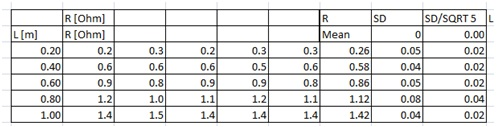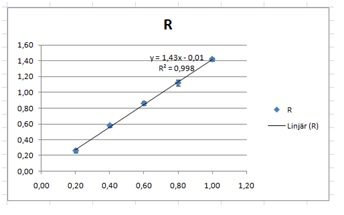# Data analysis 1, linear relations, proportionalities, Zooming in

Up a level : IB Physics
Previous page : Data analysis 1, linear relations,not a proportionality
Next page : Data analysis 1, linear relations, Zooming in, but not through the originZooming in

The data for this can be found in the Excel file analysis1 V2, in the leaf The Data, 2.

In this case described in the previous page  it was rather OK to see the how well it actually fitted, since the error bars where rather long, but sometimes really short, and then it can be really hard to find the two lines we need. Say we repeat the previous lab, but that we now have been able to find the cause of some of the errors. As we can be seen in the table below, the errors seem to be much smaller.As before we started by adding a Mean, standard deviation and standard error of the mean. Next we find a line of best fit. If we add error bars we can clearly see that we cannot clearly see the error bars in question.What we can do is to first remove the expected average, i.e. we remove  from our data. We make two new columns, L and R– Rexp.. We then plot that including error bars.  We can thus write “=I7-1.43*L7” in cell M7, and then copy it down. Those values will be very close to 0, else something is wrong.  This is what you should have now:A graph of the last two columns, including errors (from the SEM, SD/SQRT 5 column) would give us:As one can see, it is impossible to add trend lines going through the error bars without them being extremely close to each other, and it would be odd if larger variation of the data points would imply smaller errors in the final result. My guess is that the / square root of 5 trick does not work in this case. And indeed, one has to be careful about using that trick.  In this case our measuring instruments where not capable of measuring better than one part in 0.1, and that means that we cannot get better result than within ±0.05 Ω.  The trick works when we have some kind of random error on top of our measuring resolution. If we plot the graph again, but now with an error of 0.05 we get:This looks much more useful.

Yet again, given that the point goes through the origin, we add two columns:I selected the values 0.07 and –0.07 to clearly see the points.

Then we add trend lines and then we plot:Next we tweak our values to fit the error bars. One can clearly see that +0.03 and –0.06 fits the data very well.  This gives us an average of  –0.015 with an error of 0.045, or –0.02±0.05.If we add this to our previous slope, 1.43, we get 1.41±0.05, and thus that

R=(1.41±0.05)L

This is consistent with our previous result R=(1.3±0.2)LUp a level : IB Physics
Previous page : Data analysis 1, linear relations,not a proportionality
Next page : Data analysis 1, linear relations, Zooming in, but not through the originLast modified: Jan 7, 2017 @ 14:38